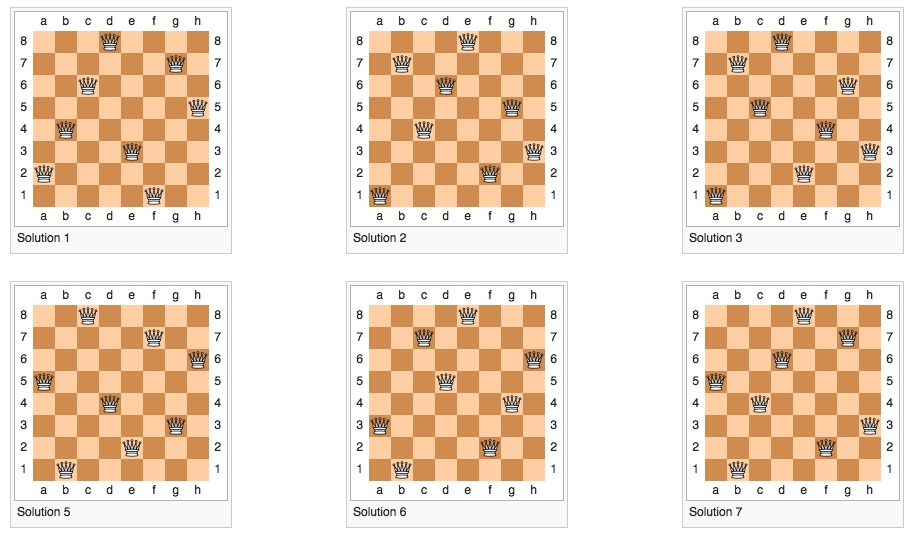# Eight queens puzzleHere’s a classic puzzle: the eight queens puzzle.

Quoted from Wikipedia:

The eight queens puzzle is the problem of placing eight chess queens on an 8×8 chessboard so that no two queens threaten each other. Thus, a solution requires that no two queens share the same row, column, or diagonal. The eight queens puzzle is an example of the more general n-queens problem of placing n queens on an n×n chessboard, where solutions exist for all natural numbers n with the exception of n=2 and n=3.

I solve puzzles from time to time to keep my motivation up and to get some exercise for my brain. But mostly for fun.

In this puzzle on a standard 8x8 chess board there are 92 solutions where no two queens threaten each other.

What could a solution in Javascript for this puzzle look like?
I’ve moved more and more into functional programming lately so this is my take on it.

``````// Solve queens puzzle.
// https://en.wikipedia.org/wiki/Eight_queens_puzzle

const doCollide = (arr1, arr2) => {
const shorter = arr1.length < arr2.length ? arr1 : arr2
const longer = arr2.length > arr1.length ? arr2 : arr1
const collisionsRemoved = shorter.filter((val, i) => shorter[i] != longer[i])
return collisionsRemoved.length != shorter.length
}

const okToAdd = (base, candidate, fn) => {
const candVector = base.reduce((last, curr, index) => {
return [].concat(last, [fn(candidate, index)])
}, [])
return !doCollide(candVector, base)
}

const upStep = (num, index) => parseInt(num) + index + 1
const downStep = (num, index) => parseInt(num) - index - 1
const levelStep = num => parseInt(num)
const getArr = (num) => Array.apply(null, Array(num))

const addQueen = (bases, candidates) => {
return bases.map((base) => {
return candidates.filter((cand) => {
return [upStep, downStep, levelStep].every((fn) => okToAdd(base, cand, fn))
}).map(val => [val].concat(base))
}).reduce((all, curr) => {
return all.concat(curr)
}, [])
}

const solve = (numTiles) => {
return getArr(numTiles).reduce((solutions, depth) => {
const candidates = getArr(numTiles).map((_, i) => i)